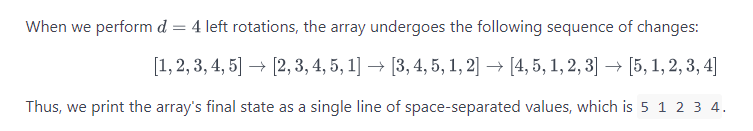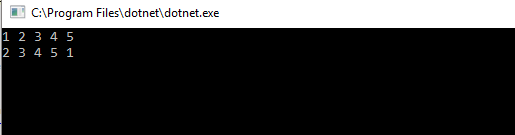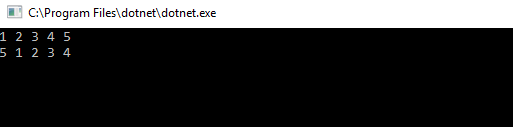# Array Left Rotation by D – C# Code

left rotation operation on an array of size n shifts each of the array’s elements 1 unit to the left. For example, if 2 left rotations are performed on array [1,2,3,4,5], then the array would become  [3,4,5,1,2].

Given an array of  n integers and a number, d , perform d left rotations on the array. Then print the updated array as a single line of space-separated integers.

Input Format

The first line contains two space-separated integers denoting the respective values of  (the number of integers) and  (the number of left rotations you must perform).
The second line contains  space-separated integers describing the respective elements of the array’s initial state.

Output Format

Print a single line of  space-separated integers denoting the final state of the array after performing  left rotations.

Sample Input

Sample Output

ExplanationCode

We can see the results for 1 and 4 rotations as follows:This site uses Akismet to reduce spam. Learn how your comment data is processed.